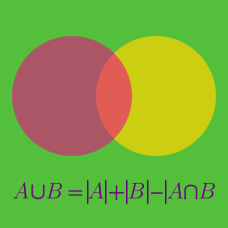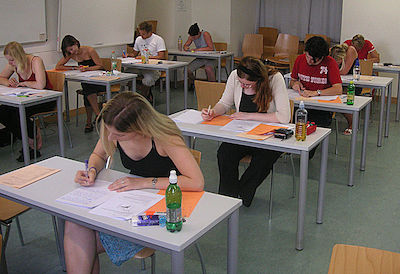Discrete Mathematics

# Principle of Inclusion and Exclusion Problem SolvingAfter a grueling nationwide examination, it was announced that
70% of students passed the Mathematics examination, and
60% of students passes the Science examination.

What is the minimum percentage of students that passed both examinations?

In a class of students, $$64\text{%}$$ of the students passed the physics exam and $$68\text{%}$$ of the students passed the math exam.

What is the minimum percentage of students in the class that passed both exams?

How many numbers in the set $$\{1,2,\ldots,100\}$$ are divisible by $$5$$ or $$7?$$

A party of $$370$$ vile creatures is marching through the woods. Each of the creatures is either an orc, a goblin, or a half-orc/half-goblin. If there are $$206$$ creatures that are at least partly goblin, and $$211$$ creatures that are at least partly orcs, how many of the creatures are half-orc/half-goblins?

Consider all $$4$$-digit positive numbers using all of the digits $$\{1, 2, 3, 4\}$$. How many of these numbers have even first or second digit?

×

Problem Loading...

Note Loading...

Set Loading...